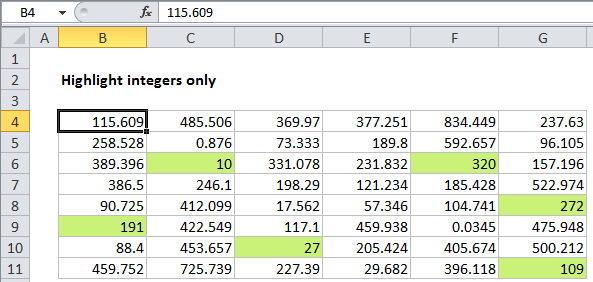## Excel Office

Excel How Tos, Tutorials, Tips & Tricks, Shortcuts

# Highlight integers only in Excel

This tutorial shows how to Highlight integers only in Excel using the example below;

## Formula

`=MOD(A1,1)=0`## Explanation

To highlight numbers that are integers, you can use a simple formula based on the MOD function. In the example shown, conditional formatting has been applied to the range B4:G11 using this formula:

`=MOD(B4,1)=0`

Note: it’s important that CF formulas be entered relative to the “active cell” in the selection, which is assumed to be B4 in this case.

Also See:   Highlight approximate match lookup conditional formatting in Excel

Once you save the rule, you’ll see only values that are integers (whole numbers) highlighted.

### How this formula works

The MOD function returns the remainder after division. With a divisor of 1, MOD will return zero for any whole number.

We use this fact to construct a simple formula that tests the result of MOD. When the result is zero (i.e. when the number is an integer) the formula returns TRUE, triggering the conditional formatting.

Also See:   Highlight cells that begin with in Excel

When the result is not zero (i.e. the number has a decimal component, and dividing by 1 leaves remainder) the formula returns FALSE.